Published: 15 November 2015

# Singular spectrum analysis and its application in Lamb wave-based damage detection

Liu Liu1
Yunju Yan2
1, 2Northwestern Polytechnical University, Xi’an, China
Corresponding Author:
Yunju Yan
Views 91

#### Abstract

This paper proposes singular spectrum analysis (SSA) based feature extraction method in Lamb wave based damage detection. SSA is used for the decomposition of the acquired Lamb wave signal into an additive set of principal components and a new universal approach for selection of the principal components is presented. The principal components which contain the least measurement noise and the most damage information are then used to detect local damage in an aluminum plate and a new approach based on the maximum likelihood analysis for damage signal decomposition is proposed. Genetic algorithm is adopted for the purpose of making the similarity between the synthetic signal and the target signal reach the maximum. The experimental result shows that the proposed method is capable of yielding accurate identified results with noisy measurement.

## 1. Introduction

Acousto-ultrasonic waves have shown great potential for damage detection in metallic and composite structures (e.g. [1-7]). This is particularly relevant to guided waves such as Lamb waves. Lamb waves are guided waves in traction-free thin plates. Plate-like structures are common in a number of aerospace, ground and sea transportation applications. Therefore Lamb waves are often used for crack monitoring in metallic structures and impact damage detection in composite structures. However, during the generation and sampling of wave signals, various types of noise are induced unavoidably, which will inevitably result in big error or even false judgment. Besides, many existing methods for extracting damage scattered signal are sensitive to external factors such as the environment and it is often difficult to extract damage scattered waves from the reflected sources since there are boundary reflected waves exist. Thus, how to reduce the noise interference and obtain a good extraction result becomes the key point for damage detection.

Singular spectrum analysis (SSA) which is based on singular value decomposition (SVD) has been used in many applications. Broomhead and King  have made the original contribution of SSA for reporting that the singular value decomposition is effective for noise reduction. This technique has been adopted in forecasting, smoothing, filtering, trend extraction , and it has been used in many research areas such as climatology [10, 11], biosciences , geology [13, 14], economics  and solar physics . Some researchers [17-19] also adopted the SSA technique in structural engineering.

In this work, a simple time series method for experimental data processing in structure damage detection using SSA is proposed. A new approach for the selection of the principal components are discussed which just need to determine the optimal components but not the unique components. In the experimental analysis, active Lamb wave technique is used for the damage detection of an aluminum plate and a selected subset of the singular values is adopted as the dynamic feature. An approach based on the maximum likelihood analysis is then proposed. Damage reflected signal and boundary reflected signal are simulated on the basis of analyzing Lamb wave propagation characteristics, and various parameters of the synthetic signal are optimized through genetic algorithm in order to make the similarity between the synthetic signal and the target signal reach the maximum.

## 2. Singular spectrum analysis

SSA is a time series analysis technique which decomposes the signal into an additive set of independent time series (principal components). In this paper SSA is applied to the response obtained by the experiment. The SSA algorithm is described below.

### 2.1. Embedding

A trajectory matrix or a lagged version is obtained during this step. Let $y=\left[{y}_{1},{y}_{2},\dots ,{y}_{N}\right]$ be a time series of length $N$, $L$ is an integer (window length) and $K$ is the number of lagged vector ($K=N-L+1$). The obtained trajectory matrix is defined as:

1
$\mathbf{Y}=\left[{Y}_{1},{Y}_{2},\dots ,{Y}_{K}\right]=\left(\begin{array}{cccc}{y}_{1}& {y}_{2}& \dots & {y}_{K}\\ {y}_{2}& {y}_{3}& \dots & {y}_{K+1}\\ ⋮& ⋮& \ddots & ⋮\\ {y}_{L}& {y}_{L+1}& \dots & {y}_{N}\end{array}\right).$

Eq. (1) shows that the trajectory matrix is a Hankel matrix since all the elements along the ‘anti-diagonals’ are the same.

### 2.2. Singular value decomposition (SVD)

In this step, the SVD of the matrix $\mathbf{Y}$ is obtained. It is further decomposed into a sum of mutually orthogonal, unit-rank, elementary matrices. Let $\mathbf{S}=\mathbf{Y}{\mathbf{Y}}^{T}$ be a $L$×$L$ matrix. Let ${\lambda }_{1}$, ${\lambda }_{2}$,…, ${\lambda }_{r}$ be the non-zero eigenvalues of $\mathbf{S}$ ($r=L$ if no eigenvalue is zero) arranged in decreasing order, and ${U}_{1}$, ${U}_{2}$,…, ${U}_{r}$ be the corresponding eigenvectors. The vectors ${V}_{j}={\mathbf{Y}}^{T}\left({U}_{j}/\sqrt{{\lambda }_{j}}\right)$ for $j=$ 1, 2,…, $r$ is constructed.

The SVD of the trajectory matrix $\mathbf{Y}$ can be written as:

2
$\mathbf{Y}={\mathbf{Y}}_{1}+{\mathbf{Y}}_{2}+...+{\mathbf{Y}}_{r},$

where ${\mathbf{Y}}_{j}=\sqrt{{\lambda }_{j}}{U}_{j}{V}_{j}^{T}$.

The obtained $L$ numbers of singular value are the square roots of the eigenvalues of matrix $\mathbf{S}$. The plot of the singular value in decreasing order is known as singular spectrum, and thus gives the method its name.

### 2.3. Diagonal averaging

Each matrix of Eq. (2) is transformed into a new time series of length $N$ by applying a linear transformation known as diagonal averaging. The diagonal averaging algorithm is as follows:

Since $\mathbf{Y}$ is an $L$×$K$ matrix with elements ${y}_{ij}$, 1 $\le i\le L$, 1 $\le j\le K$. Set ${L}^{\mathrm{*}}=\mathrm{min}\left(L,K\right)$, ${K}^{\mathrm{*}}=\mathrm{max}\left(L,K\right)$ and $N=L+K-1$. Let ${y}_{ij}^{\mathrm{*}}={y}_{ij}$ if $L and ${y}_{ij}^{\mathrm{*}}={y}_{ji}$ otherwise. By averaging the diagonal the matrix $\mathbf{Y}$ is transferred into the series ${y}_{1}$,…, ${y}_{N}$ using the formula:

3
${y}_{k}=\left\{\begin{array}{ll}\frac{1}{k}{\sum }_{m=1}^{k}{y}_{m,k-m+1}^{\mathrm{*}},& 1\le k<{L}^{\mathrm{*}},\\ \frac{1}{{L}^{\mathrm{*}}}{\sum }_{m=1}^{{L}^{\mathrm{*}}}{y}_{m,k-m+1}^{\mathrm{*}},& {L}^{\mathrm{*}}\le k\le {K}^{\mathrm{*}},\\ \frac{1}{N-k+1}{\sum }_{m=k-{K}^{\mathrm{*}}+1}^{N-{K}^{\mathrm{*}}+1}{y}_{m,k-m+1\mathrm{}}^{\mathrm{*}},& {K}^{\mathrm{*}}

The diagonal averaging procedure is applied to all the selected matrix components $\left({\mathbf{Y}}_{1}$, ${\mathbf{Y}}_{2}$,…, ${\mathbf{Y}}_{r}\right)$ to form Hankel matrices $\left({\stackrel{-}{\mathbf{Y}}}_{1}$, ${\stackrel{-}{\mathbf{Y}}}_{2}$,…, ${\stackrel{-}{\mathbf{Y}}}_{r}\right)$. Each Hankel matrix determines a time series that is recognized as the projection of the original signal in the corresponding direction determined by the corresponding eigentriple. Then the sum of all selected projected time series component represents the reconstructed signal.

### 2.4. Parameter selection

It should be mentioned that two parameters are required in SSA: the window length $L$ and the number of reconstructed components $r$. A detailed description of parameter selection is presented in Golyandina et al. . Values for $L$ and $r$ could be defined using information provided by the time series under study or through additional indices. The general rule is to select $L=N/2$, which is also the value used in this paper. The dimension of the selected components, which is defined as $r$, is very important. In fact the “selection” of useful signals with SSA is still under studied with no unified approach. However, by the data structure of Hankel matrix it can be seen that the next row vector is just lagging one data point compare to the front row vector. Therefore the adjacent two lines of the noise-free Hankel matrix (in a morbid form) will be highly correlated, and the rank $r$ is much smaller than min ($m$, $n$). The number of non-zero singular value is equal to the rank of the matrix according to the theory of SVD, which means only the first $r$ singular values are non-zero, while the rest are substantially zero. And there will be a curve exists at the turning point.

For the noise constructed Hankel matrix, the autocorrelation function of noise sequence $\zeta \left(i\right)$ is as follows:

4
${R}_{\xi }\left(\tau \right)={b}^{2}\delta \left(\tau \right),$

where $b$ is standard deviation of $\zeta \left(i\right)$, $\delta \left(\tau \right)$ is Kronecker function:

5
$\delta \left(\tau \right)=\left\{\begin{array}{l}1,\mathrm{}\mathrm{}\mathrm{}\mathrm{}\tau =0,\\ 0,\mathrm{}\mathrm{}\mathrm{}\mathrm{}\tau \ne 0.\mathrm{}\end{array}\right\$

Eq. (4) and Eq. (5) show that there is no correlation between the two adjacent rows of the noise constructed Hankel matrix, the matrix is benign with rank $r=\mathrm{m}\mathrm{i}\mathrm{n}\left(m,n\right)$. There will be no turning point exists in the singular value curve for the noise-only condition. Thus, the turning point of the singular value curve can be the distinction of real signal and noise involved signal. At this turning point energy of the real signal is more concentrated, while the noise energy is relatively dispersed. It can be concluded that there will be a “peak” (not unique) at the boundary between signal and noise, and noise makes main contribution after that “peak”. Singular value differential spectrum value is then defined as:

6
$\rho \left(i\right)=\left|{\lambda }_{i+1}-{\lambda }_{i}\right|,\mathrm{}\mathrm{}\mathrm{}\left(i=1,\mathrm{}2,\dots ,r\right).$

By Eq. (6) we can select the critical point $r$ (also not unique) to be the reconstructed components.

## 3. Damage detection for an aluminum plate that integrating SSA and Lamb waves

### 3.1. Modeling Lamb wave for damage detection

This subsection is mainly about the introduction of active Lamb wave in structure damage detection. Lamb waves are firstly inspired by piezoelectric ceramics and narrow band excitation is applied here which has a form as bellows:

7
$f\left(t\right)=A\left[H\left(t\right)-H\left(t-\frac{n}{{f}_{c}}\right)\right]\left(1-\mathrm{c}\mathrm{o}\mathrm{s}\frac{2\pi {f}_{c}t}{n}\right)\mathrm{s}\mathrm{i}\mathrm{n}2\pi {f}_{c}t,$

where $n$ is the peak number, ${f}_{c}$ is the center frequency, $A$ is the amplitude modulation and $H$ is the Heaviside step function.

The inspired field quantity is expressed as $u\left(x,t\right)$, where $x$ presents the propagation distance and $t$ presents the propagation time. If one point of $u\left(x,t\right)$ and the propagation characteristics of Lamb waves are known, then any point of $u\left(x,t\right)$ may be obtained by the phase shift of each frequency component. And $u\left(x,t\right)$ can be expressed as :

8
$u\left(x,t\right)={\int }_{-\mathrm{\infty }}^{\mathrm{\infty }}F\left(\omega \right){e}^{i\left(k\left(\omega \right)x-\omega t\right)}d\omega ,$

where $k\left(\omega \right)$ is the wave number, $\omega$ is the angular frequency and $F\left(\omega \right)$ is the Fourier transform of $f\left(t\right)$.

During the propagation the inspired signal will encounter reflector (such as damage spot or boundary) and the reflected signal $g\left(t\right)$ is typically superimposed by different reflected sources. The reflected signal to the sensor which is going across the $j$th reflected source can be expressed as:

9
${g}_{j}\left(t\right)={\int }_{-\mathrm{\infty }}^{\mathrm{\infty }}{A}_{j}\left(\omega \right)F\left(\omega \right){e}^{i\left(k\left(\omega \right)\left({x}_{j}+{d}_{j}\right)-\omega t\right)}d\omega ,$

where ${d}_{j}$ is the distance between actuator and reflector, ${x}_{j}$ is the distance between reflector and sensor, $A{}_{j}{}^{\mathrm{}}\left(\omega \right)$ is the reflection coefficient.

The propagation distance from the actuator to the sensor is defined as ${l}_{j}$ and:

10
${l}_{j}={x}_{j}+{d}_{j}.$

Then Eq. (9) can be expressed as:

11
${g}_{j}\left(t\right)={\int }_{-\mathrm{\infty }}^{\mathrm{\infty }}{A}_{j}\left(\omega \right)F\left(\omega \right){e}^{i\left(k\left(\omega \right){l}_{j}-\omega t\right)}d\omega .$

Fig. 1Schematic diagram of detection principle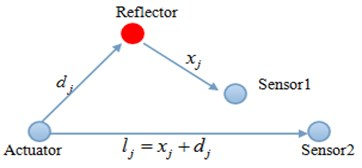Eq. (9) and Eq. (11) show that signal from the $j$th reflected source ${g}_{j}\left(t\right)$ can be considered as the propagation signal from the actuator to another sensor (see Fig. 1).

### 3.2. Damage detection based on the maximum similarity analysis

In this subsection the maximum similarity analysis method is introduced. This method is based on the priori knowledge as below:

1. Dispersion characteristics of the propagated Lamb waves are obtained from the material properties;

2. Direct wave arrival time is known when structural model dimensions and actuator/sensor arrangement are determined;

3. Reflected signal is typically superimposed by different reflected sources and it can be determined by the form of the inspired signal and propagation distance.

It is assumed that there are totally $n$ reflected sources and all of them (damage scattered waves or boundary reflected waves) can be determined by Eq. (11), the synthetic signal that consists of damage scattered waves and boundary reflected waves is further obtained as:

12
$G\left(t\right)={\sum }_{n}{a}_{n}{g}_{n}\left(t\right)={\sum }_{n}{a}_{n}{\int }_{-\mathrm{\infty }}^{\mathrm{\infty }}{A}_{n}\left(\omega \right)F\left(\omega \right){e}^{i\left(k\left(\omega \right){l}_{n}-\omega t\right)}d\omega ,$

where ${a}_{n}$ is the control factor of the amplitude.

Then the extraction of damage scattered signal can be converted to the optimization problem: Parameter optimization (${a}_{n}$, ${l}_{n}$ and $n$) that base on the Genetic Algorithms (GA). Cosine method  is applied to be the similarity index to measure the degree of coincidence between the synthetic signal and the target signal, the formula is as follows:

13
$\mathrm{c}\mathrm{o}\mathrm{s}\left(x,y\right)=\frac{{\sum }_{i}\left({x}_{i}{y}_{i}\right)}{\sqrt{{\sum }_{i}\left({x}_{i}^{2}\right){\sum }_{i}\left({y}_{i}^{2}\right)}},$

where $x$ presents the synthetic signal and $y$ presents the target signal. The fitness function is as follows:

14
$Z=1-\mathrm{c}\mathrm{o}\mathrm{s}\left(x,y\right)=1-\frac{{\sum }_{i}\left({x}_{i}{y}_{i}\right)}{\sqrt{{\sum }_{i}\left({x}_{i}^{2}\right){\sum }_{i}\left({y}_{i}^{2}\right)}}.$

The two signals have a better similarity when fitness function value is closer to zero. Fig. 2 shows the main process of the maximum similarity analysis that based on GA.

Fig. 2Block diagram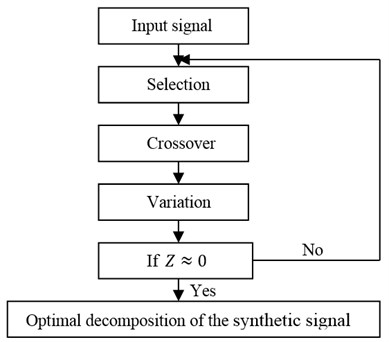### 3.3. Damage location

Time of Flight  method is used for damage location. The actuator and sensor can be regarded as two focus of an ellipse, which means the ellipse can be determined. However, damage can’t be located if only one ellipse is known. So another sensor is added for obtaining a second ellipse and the intersection of the two ellipses is the exact damage location.

### 3.4. Experiment analysis

Test device and specimen are shown in Fig. 3 and Fig. 4, including Sonox P5 circular piezoelectric plate (diameter is 6 mm), RIGOL DG1022 Arbitrary Waveform Generator, HVPA05 amplifier, Tektronix TDS2014C oscilloscopes and 2024-T3 aluminum specimen (dimension is 600 mm×600 mm×1 mm). The boundary-free aluminum plate is supported by foam and the material parameters are shown in Table 1. Three piezoelectric sheets are pasted with conductive silver colloid. PZT A is actuator, PZT B and PZT C is sensor. A transparent hole (diameter is 5 mm) is represented for the damage, five-cycle sine signal (modulated with Hanning window) is adopted as the inspired signal, with the center frequency of 300 KHz and the inspired voltage peak is 20 V. In addition, TDS2014C digital oscilloscope is set to 256 times average automatically processing in order to improve the stability and signal to noise ratio, and trigger interval is set to 1 second to ensure that the last inspiration and reflection disappear completely. Equipment and each piezoelectric sheet are accessed to the ground to avoid electromagnetic interference wave packet.

Fig. 3Test device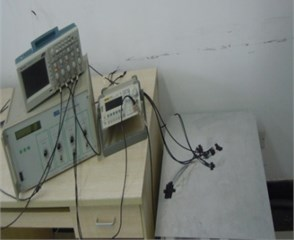Fig. 4Test specimen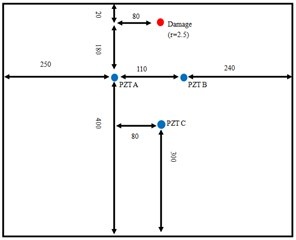Table 1Material parameters of the aluminum sheet (cl: longitudinal wave velocity; ct: shear wave velocity)

 $E$ (GPa) $\rho$ (kg/m3) $h$ (mm) ${c}_{l}$ (m/s) ${c}_{t}$ (m/s) 72.4 2780 1 6211.8 3129

Original response signal of path AB is shown in Fig. 5. SSA technique is applied for the original signal and the singular value differential spectrum is shown in Fig. 6. In Fig. 6 “peak” exist when $r$ is equal to 2, 4, 6, 8, 9, 11, 13 and 19. The reconstructed results of these components are plotted in Fig. 6 respectively. The first 19 components reconstructed result is a very accurate approximation to the real response on comparison of Fig. 5 and Fig. 7. Since no aliasing happens in direct wave so the target signal can be simplified as the dashed frame part of Fig. 7(h).

Fig. 5Damage signal captured by PZT B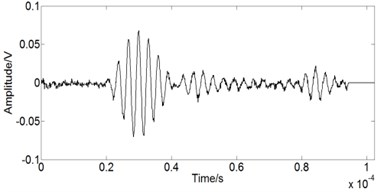Fig. 6Singular value differential spectrum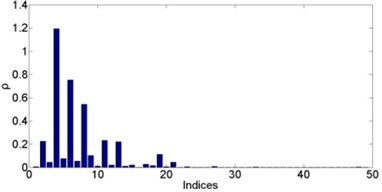Maximum similarity analysis that based on GA is applied for the optimization of the target signal and the optimization results are shown in Table 2. Here $n$ presents the number of reflected waves (damage scattered wave or boundary reflected waves). The accurate propagation distance $l$ and propagation time $t$ of the boundary reflected wave can be obtained by the position of the sensor and the boundary (see Table 3).

Fig. 7Reconstructed responses with different combinations of components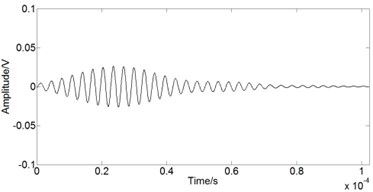a) First 2 components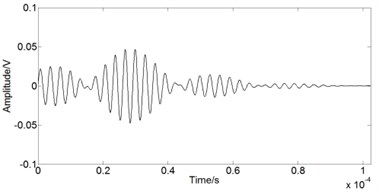b) First 4 components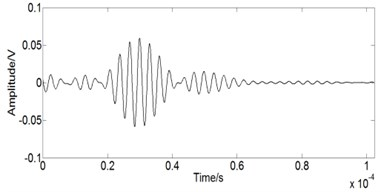c) First 6 components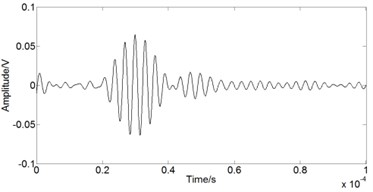d) First 8 components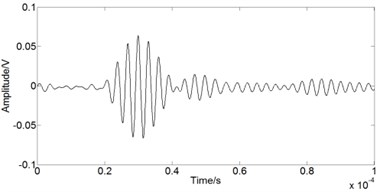e) First 9 components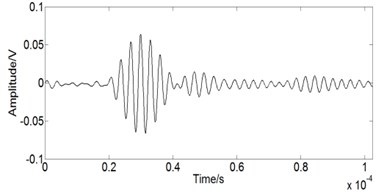f) First 11 components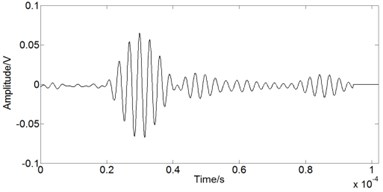g) First 13 components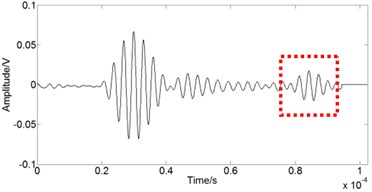h) First 19 components

Table 2Optimization results by GA

 $n$ $Z$ ${l}_{n}$ (m) ${a}_{n}$ 1 0.342 0.410 0.440 2 0.099 0.380, 0.416 –0.425, 0.434 3 0.128 0.415, 0.379, 0.417 0.574, 1.030, 1.433 4 0.185 0.223, 0.406, 0.440, 0.408 0.078, 0.916, 0.440, 0.408 5 0.068 0.393, 0.432, 0.409, 0.446, 0.388 1.678, 0.538, 0.961, 0.946, 2.559

Table 3Boundary path

 Parameter Upper boundary Lower boundary Left boundary Right boundary $l$ (m) 0.415 0.808 0.66 0.59 $t$ (10-5 s) 7.701 14.993 12.254 10.954

Table 3 shows the accurate propagation distance $l$ is equal to 0.415, and 0.415 also appears in Table 3 when $n=$ 2 and $n=$ 3. Since there will be only one damage scattered wave and the rest are boundary reflected waves, optimization results match the known conditions best when $n=$ 2. So the synthetic signal contains two waves: damage scattered wave and upper boundary reflected wave. The time domain waveform is shown in Fig. 8. Fig. 9 shows the comparison of synthetic signal and target signal. In addition, Fig. 4 shows that the accurate propagation of damage scattered wave ${l}_{accurate}$ is equal to 0.3795 m, while the optimization results fit it very well (${l}_{calculate}=$0.380, see Table 2).

Fig. 8Reflected signal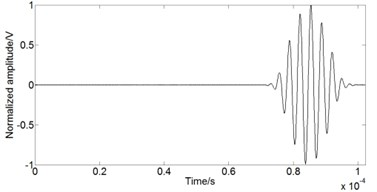a) Boundary reflected wave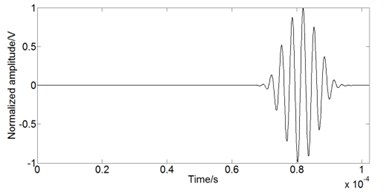b) Damage scattered wave

Fig. 9Comparison of synthetic signal and target signal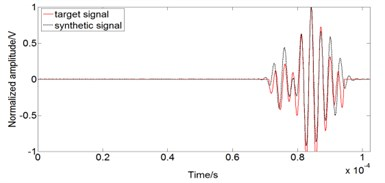In order to obtain the exact damage location another path AC is analyzed. Fig. 10 shows the original response signal of path AC. SSA technique is also applied for the original signal and the singular value differential spectrum is shown in Fig. 11. “Peak” exists when $r$ is equal to 2, 4, 6, 8, 10, 11, 13, 15, 17, 19, 21 and 24. The reconstructed results of these components are plotted in Fig. 12 respectively. The first 21 components reconstructed result is a very accurate approximation to the real response on comparison of Fig. 10 and Fig. 12. The same optimization analysis is conducted and Table 4 shows the damage localization results. The identification results show that the damage location can be identified accurately with small positive errors when the best reconstructed components are selected. Fig. 13 shows the comparison of the actual position and the ellipse orientation position.

Fig. 10Damage signal captured by PZT C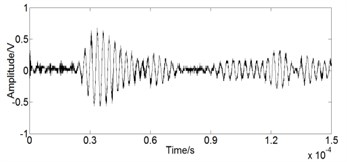Fig. 11Singular value differential spectrum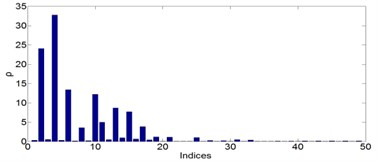Table 4Damage localization results

 Path ${\left(l\right)}_{actual}$ / m ${\left(l\right)}_{calculate}$ / m Actual position / m Calculate position / m Error / m AB 0.3795 0.3810 (0.33, 0.58) (0.3321, 0.5801) 0.0021 AC 0.4770 0.4780

Fig. 12Reconstructed responses with different combinations of components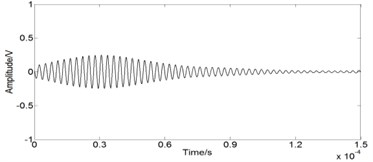a) First 2 components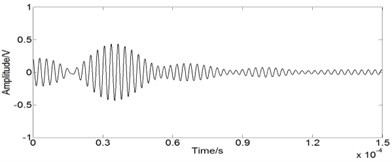b) First 4 components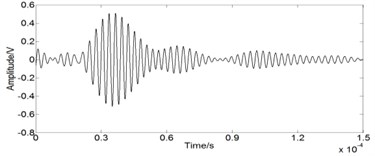c) First 6 components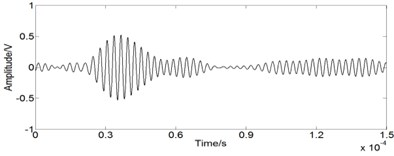d) First 8 components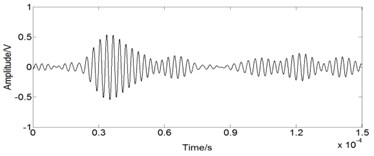e) First 10 components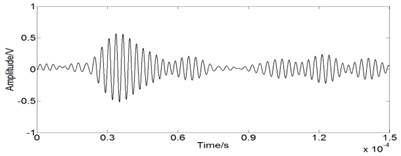f) First 11 components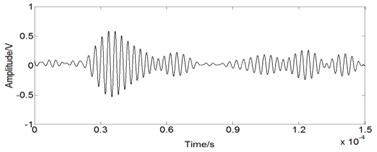g) First 13 components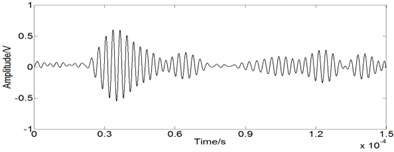h) First 15 components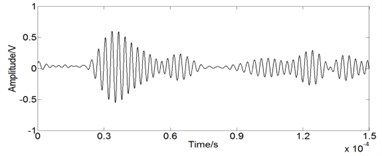i) First 17 components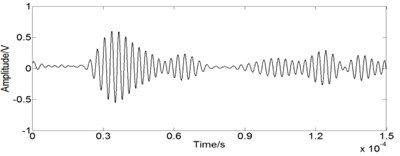j) First 19 components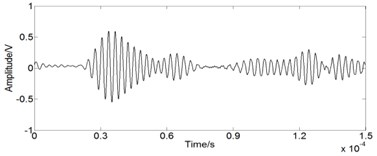k) First 21 components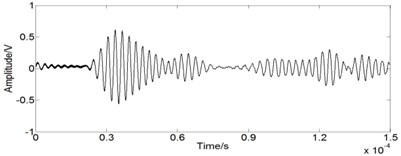l) First 24 components

## 4. Conclusion

The presented work discussed SSA technique in experimental data processing and a new damage detection method based on SSA and Lamb wave was also proposed. Experimental response was decomposed with the SSA technique and a new approach for selection of the principal components was employed. In the experimental analysis, Lamb wave was applied for damage detection and the maximum likelihood theory based on Genetic Algorithm was generalized. Time of Flight method was used for damage location. It shows that the features obtained from the principal components corresponding to singular value numbers are useful for damage detection. The correspondence between the real damage and the calculated damage has also been shown. The experimental results demonstrate that the proposed damage detection method is simple, noise tolerant and efficient.

Fig. 13Comparison of the actual position and the ellipse orientation position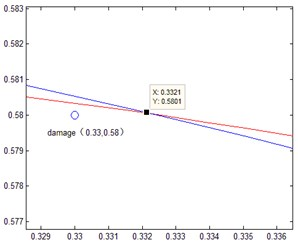a) Ellipse orientation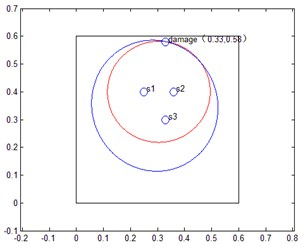b) Ellipse intersection

15 April 2015
Accepted
28 July 2015
Published
15 November 2015
SUBJECTS
Fault diagnosis based on vibration signal analysis
Keywords
singular spectrum analysis
damage detection
Lamb wave
genetic algorithm
Acknowledgements

The authors would like to thank for the supports by the National Natural Science Foundation of China under the Grant 50875212 and Specialized Research Fund (priority development area) for the Doctoral Program of Higher Education of China under the Grant 20126102130004. This work is also supported by the Chinese Scholarship Council for the visiting scholar program at the University of Michigan.